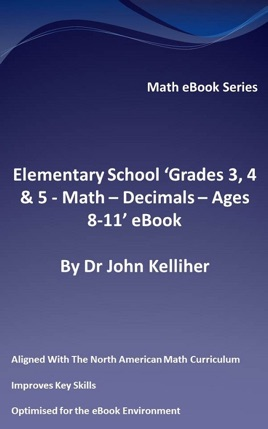• \$2.99

## Publisher Description

This eBook introduces decimals, the importance of the decimal point and its position within a number, reviews decimal notation, locates decimals on a number line, converts fractions to decimals, considers multiplying and dividing decimals by 10 and 100 respectively, and considers rounding decimals to the nearest whole number, tenth, and hundredth.This eBook is part of our range of Grades 3, 4 & 5 math eBooks that are aligned with the North American math curriculum.Our Grade 3, 4 & 5 math eBooks comprise three principle sections. These are, notably:•Number and Algebra•Shape, Space and Measure•Handling DataIn addition, there exists for Grades 3, 4 & 5 a Publications Guide eBook, a Mental Math eBook and a Times Table Practice eBook. We also produce a combined Grades 1 & 2 and Grades 3, 4 & 5 Times Table Practice eBook.Our math eBooks are produced such as that as well as a Publications Guide, and three principle publications corresponding to the principle sections (Number and Algebra, Shape, Space and Measures as well as Handling Data) there are individual modules produced within each principle section which are published as eBooks.Decimals is a module within the Number and Algebra principle section our Key Stage 2 (KS2) publications. It is one module out of a total of eight modules in that principle section, the others being:•Counting Practice•Number Patterns and Sequences•Integers•Fractions, Percentages and ratio•Addition and Subtraction Practice•Multiplication and Division Practice•AlgebraAfter studying Elementary School 'Grades 3, 4 & 5 - Math – Decimals - Ages 8-11' eBook your child or the student should be confidently able to:-understand and use decimal notation for tenths, hundredths and thousandths as for example dealing with money and converting lengths, such as converting 1.74 meters to centimeters as well to meters.-locate decimals on a number line as well as order a set of numbers or measurements.-round a number to zero, one or two decimal places.-convert between centimeters, millimeters and meters.-convert between millimeters and meters as well as between meters and kilometers, correctly applying the methods and explaining their reasoning.